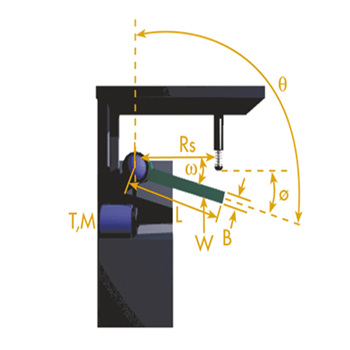# Buffersfor industrial Applications

By joining forces with an experienced Asian partner, Furka Reibbeläge AG is able to deliver high quality buffers based on many years of manufacturing, development and testing know-how.• Amount: pcs
Cycles: /hr
Weight (W): kg
Length (L): m
Thickness (B): m
Torque (T): Nm
Angle of rotation at impact (ᴓ): deg
Starting point from true vertical (θ): deg
• Amount: pcs
Cycles: /hr
Weight (W): kg
Length (L): m
Thickness (B): m
Torque (T): Nm
Angle of rotation at impact (ᴓ): deg
Starting point from true vertical (θ): deg
• Amount: pcs
Cycles: /hr
Torque (T): Nm
Angle of rotation at impact (ᴓ): deg
Starting point from true vertical (θ): deg
• Amount: pcs
Cycles: /hr
Torque (T): Nm
Angle of rotation at impact (ᴓ): deg
Starting point from true vertical (θ): deg
• Amount: pcs
Cycles: /hr
Weight of Table: kg
Rotary Table Diameter (d): m
Torque (T): Nm
• Amount: pcs
Cycles: /hr
Weight (W): kg
Length (L): m
Thickness (B): m
Torque (T): Nm
• Amount: pcs
Cycles: /hr
Torque (T): Nm
• Amount:
pcs
Cycles: /hr
Weight: kg
Height: m
Angle of Incline (α): deg
• Amount: pcs
Cycles: /hr
Weight: kg
Velocity: m/s
Drive Force: N
• Amount: pcs
Cycles: /hr
Weight: kg
Velocity: m/s
Drive Force: N
• Amount: pcs
Cycles: /hr
Weight: kg
Velocity: m/s
Motor Rating: kW
• Amount: pcs
Cycles: /hr
Weight: kg
Velocity: m/s
Motor Rating: kW
• Amount: pcs
Cycles: /hr
Weight: kg
Velocity: m/s
Number of Propelling Cylinders: pcs
Cylinder Bore Dia: mm
Pressure: Bar
• Amount: pcs
Cycles: /hr
Weight: kg
Velocity: m/s
Number of Propelling Cylinders: pcs
Cylinder Bore Dia: mm
Pressure: Bar
• Amount: pcs
Cycles: /hr
Weight: kg
Height: m
• Amount: pcs
Cycles: /hr
Weight: kg
Velocity: m/s
Number of Propelling Cylinders: pcs
Cylinder Bore Dia: mm
Pressure: Bar
• Amount: pcs
Cycles: /hr
Weight: kg
Velocity: m/s
Drive Force: N
• Amount: pcs
Cycles: /hr
Weight: kg
Velocity: m/s
Motor Rating: kW
• Amount: pcs
Cycles: /hr
Weight: kg
Velocity: m/s
Option Type Model Stroke Thread Length Max Energy (Nm/c) % of Max Energy Nm/c (Et) Max Energy (Nm/h) % of Max Energy Nm/h (EtC) Max Shock Force (N) Max Propelling Force (N)
•  Your Input Number of Shock Absorbers (pcs) Cycles (/hr) Weight (W) (kg) Lenght (L) (m) Thickness (B) (m) Angular Velocity (ω) (rad/s) Torque (T) (Nm) Mounting Radius (Rs) (m) Angle of rotation at impact (ᴓ) ( deg) Starting point from true vertical (θ) (deg)
•  Your Input Number of Shock Absorbers (pcs) Cycles (/hr) Weight (W) (kg) Lenght (L) (m) Thickness (B) (m) Angular Velocity (ω) (rad/s) Torque (T) (Nm) Mounting Radius (Rs) (m) Angle of rotation at impact (ᴓ) ( deg) Starting point from true vertical (θ) (deg)
•  Your Input Number of Shock Absorbers (pcs) Cycles (/hr) Installed Load (W1) (kg) Radius of Gyration (K) (m) Angular Velocity (ω) (m) Torque (T) (rad/s) Mounting Radius (Rs) (Nm) Angle of rotation at impact (ᴓ) (M) Starting point from true vertical (θ) (deg)
•  Your Input Number of Shock Absorbers (pcs) Cycles (/hr) Installed Load (W1) (kg) Radius of Gyration (K) (m) Angular Velocity (ω) (rad/s) Torque (T) (rad/Nm) Mounting Radius (Rs) (m) Angle of rotation at impact (ᴓ) (deg) Starting point from true vertical (θ) (deg)
•  Your Input Number of Shock Absorbers (pcs) Cycles (/hr) Weight of Table (kg) Installed Load (W1) (kg) Radius of Gyration (K) (m) Rotary Table Diameter (d) (m) Angular Velocity (ω) (rad/s) Torque (T) (Nm) Mounting Radius (Rs) (m)
•  Your Input Number of Shock Absorbers (pcs) Cycles (/hr) Installed Load (W1) (kg) Radius of Gyration (K) (m) Angular Velocity (rad/s) Torque (T) (Nm) Mounting Radius (Rs) (m)
•  Your Input Number of Shock Absorbers (pcs) Cycles (/hr) Weight (W) (kg) Lenght (L) (m) Thickness (B) (m) Angular Velocity (ω) (rad/s) Torque (T) (Nm) Mounting Radius (Rs) (m)
•  Your Input Number of Shock Absorbers (pcs) Cycles (/hr) Weight (kg) Height (m) Angle of Incline α(deg)
•  Your Input Number of Shock Absorbers (pcs) Cycles (/hr) Weight (kg) Velocity (m/s) Drive Force (N)
•  Your Input Number of Shock Absorbers (pcs) Cycles (/hr) Weight (kg) Velocity (m/s) Drive Force (N)
•  Your Input Number of Shock Absorbers (pcs) Cycles (/hr) Weight (kg) Velocity (m/s) Motor Rating (kW)
•  Your Input Number of Shock Absorbers (pcs) Cycles (/hr) Weight (kg) Velocity (m/s) Motor Rating (kW)
•  Your Input Number of Shock Absorbers (pcs) Cycles (/hr) Weight (kg) Velocity (m/s) Number of Propelling Cylinders (pcs) Cylinder Bore Dia (mm) Pressure (Bar)
•  Your Input Number of Shock Absorbers (pcs) Cycles (/hr) Weight (kg) Velocity (m/s) Number of Propelling Cylinders (pcs) Cylinder Bore Dia (mm) Pressure (Bar)
•  Your Input Number of Shock Absorbers (pcs) Cycles (/hr) Weight (kg) Height (m)
•  Your Input Number of Shock Absorbers (pcs) Cycles (/hr) Weight (kg) Velocity (m/s) Number of Propelling Cylinders (pcs) Cylinder Bore Dia (mm) Pressure (Bar)
•  Your Input Number of Shock Absorbers (pcs) Cycles (/hr) Weight (kg) Velocity (m/s) Drive Force (N)
•  Your Input Number of Shock Absorbers (pcs) Cycles (/hr) Weight (kg) Velocity (m/s) Motor Rating (kW)
•  Your Input Number of Shock Absorbers (pcs) Cycles (/hr) Weight (kg) Velocity (m/s)
•  Ca Kinetic Energy (Nm) Work Energy (Nm) Total Energy (Nm/c) Total Energy per Hour (Nm/h) Drive Force (N) G Load Impact Velocity (m/s)
 Technical Data Model Type Stroke(mm) Max Energy (Nm/c) % of Max Energy Nm/c (Et) Max Energy (Nm/h) % of Max Energy Nm/h (EtC) Length (mm) Thread Max Shock Force (N) Max Propelling Force (N)